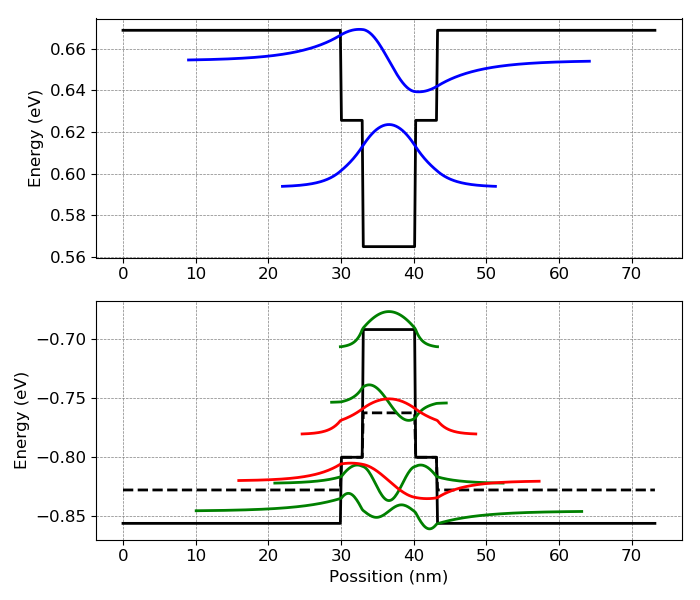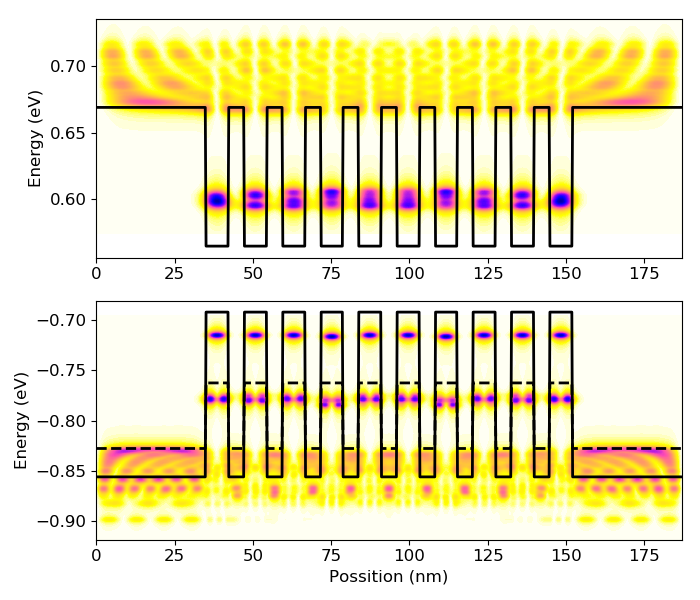# Setting up the options for and defining custom materials¶```from solcore import si, material
from solcore.structure import Layer, Structure
import solcore.quantum_mechanics as QM

# First we create the materials we need
bulk = material("GaAs")(T=293, strained=False)
barrier = material("GaAsP")(T=293, P=0.1, strained=True)

# As well as some of the layers
top_layer = Layer(width=si("30nm"), material=barrier)
inter = Layer(width=si("3nm"), material=bulk)
barrier_layer = Layer(width=si("5nm"), material=barrier)
bottom_layer = top_layer

# We create the QW material at the given composition
QW = material("InGaAs")(T=293, In=0.15, strained=True)

# And the layer
well_layer = Layer(width=si("7.2nm"), material=QW)

# The following lines create the QW structure, with different number of QWs and interlayers. Indicating the substrate
# material with the keyword "substrate" is essential in order to calculate correctly the strain.

# A single QW with interlayers
test_structure_1 = Structure([top_layer, inter, well_layer, inter, bottom_layer], substrate=bulk)
output_1 = QM.schrodinger(test_structure_1, quasiconfined=0, graphtype='potentials', num_eigenvalues=20, show=True)

# 10 QWs without interlayers
test_structure_2 = Structure([top_layer, barrier_layer] + 10 * [well_layer, barrier_layer] + [bottom_layer],
substrate=bulk)
output_2 = QM.schrodinger(test_structure_2, quasiconfined=0.05, graphtype='potentialsLDOS', num_eigenvalues=200,
show=True)
```